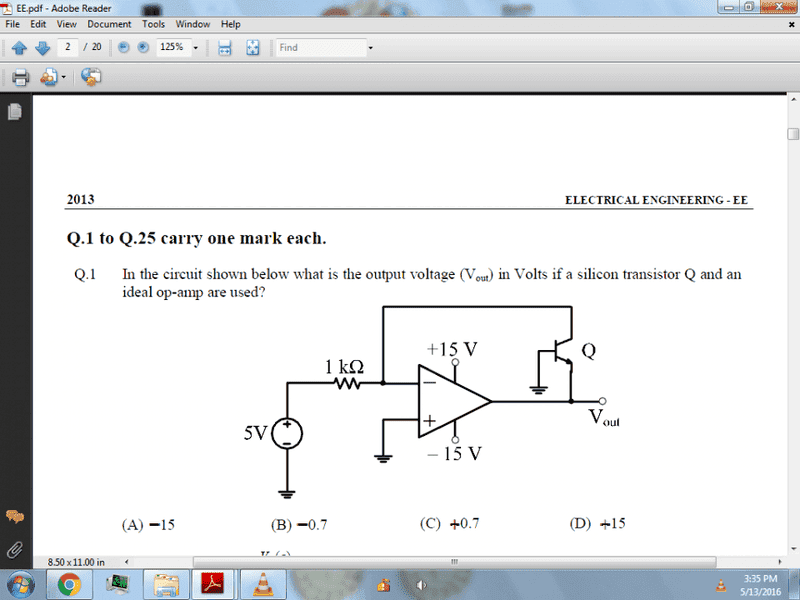# What is this circuit for?

• Engineering
• ENE
In summary, the circuit is a transistor with a feedback loop. It is used to create a constant current source.

#### ENE

Thread moved from the technical forums, so no HH Template is shown.
Hello,
What is this circuit working for?I'm pretty sure this circuit has no practical use whatever. There will be no feedback if the output ever gets positive

•ENE
I have used op amp and comprator like 741 and L339.
I don't understand the circuit Q npn.

ENE said:
I have used op amp and comprator like 741 and L339.
I don't understand the circuit Q npn.

It is a transistor. But I agree with willem2 that the circuit looks very odd and does not serve a practical purpose, I can't see why you would put a transistor in the feedback loop of an op-amp; especially not with the base grounded(!)
Hence, I don't think the circuit will do anything useful but that does not mean that you can't answer the question.

For you naysayers it is most likely a log amplifier.

•collinsmark
If a load is connected between Vout and -15V then the circuit acts as a constant current (5mA) source for resistive loads of up to 3kΩ.
This configuration is the basis of constant current sources using op amps.

It looks like it could serve a number of functions.
(a) Over a range of positive input, the output voltage will be a logarithmic function of the input voltage [at fixed temperature].

(b) Or if you maintain the input voltage fixed, the output will be a linear function of temperature of the transistor probe.

(c) Or it could simply be an analog comparator, with output of -0.7V or +15V.

•cnh1995

How to calculate it?

I didn't realize that you are asking for help with the question in your post, I thought you were asking something else, viz., what might this circuit be used for.

In operation, either the transistor will be functioning as a transistor, or it won't be. If it is not functioning as a transistor then it is "off". For the transistor to be functioning as a transistor, describe the voltages around the circuit you will definitely expect to see.

•ENE
In operation, either the transistor will be functioning as a transistor, or it won't be. If it is not functioning as a transistor then it is "off".
let the transistor be off so open circuit for feed back.
so, +ve pin to 0V
and -Ve pin to 5V
that mean it should be -15V?
how to do?

ENE said:
let the transistor be off so open circuit for feed back.
so, +ve pin to 0V
and -Ve pin to 5V
that mean it should be -15V?
Now using the result that Vout = -15V does this confirm your premise that Q is "off"?

NO, the question same as above i am guessing that it will be -15V when BJT is open.
no idea when it will work.

If Vout = -15V will this turn the transistor "off"? Look at the voltages that this type of transistor requires for it to be on or off.

The BJT is npn it will get more -ve with respect to base for turing on it want 0.7V

ENE said:
The BJT is npn it will get more -ve with respect to base for turing on it want 0.7V
We need a yes or no answer to the question: Is it possible in this circuit for Vout = -15V to be keeping the transistor off?

If these 2 conditions can coexist then that is how it must be. If these conditions cannot coexist, then you need to make a different initial assumption.

If you look at the input side of the amplifier the 5V DC with 1kΩ resistor will make a current of 1mA flow towards the inverting input. The current cannot flow into the op-amp and will therefore flow by the feedback link towards the output. It will be the collector current of the transistor.
Can you see what the output voltage will be?

lychette said:
If a load is connected between Vout and -15V then the circuit acts as a constant current (5mA) source for resistive loads of up to 3kΩ.
This is not correct.

Averagesupernova said:
For you naysayers it is most likely a log amplifier.
Yes, but not a good one.
Vout = VT ln{(Vin/Rin)/is} assuming high beta of transistor. But is = saturation current which varies with temperature & device to device within the same family. VT = kT/q, k = bolzmann constant, T = kelvin temp., q = electronic charge. It's a poor indicator of temperature also since again is = is(T).

•Averagesupernova